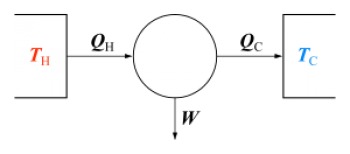# First law of thermodynamicsThe first law of thermodynamics, also called by extension, the law of conservation of energy, is a fundamental assumption of the theory of thermodynamics.

The first law of thermodynamics is a formulation of the principle of conservation of energy and states that:

"The internal energy of an isolated thermodynamic system is constant."

A thermodynamic universe, which consists of the system and its environment, is an isolated system. Energy is not created or destroyed, but is transformed from one form to another: energy can be transferred through the exchange of heat (heat energy) and work.

In the most general and simplest form, the first law of thermodynamics can be described by saying that there is a function of the thermodynamic coordinates of a system, called internal energy U. The variations of this internal energy generate the exchanges of energy of the system with the environment that surrounds it. This process characterizes the thermodynamic transformations between two equilibrium states of the system, according to which the internal energy is a function of the state. During a transformation, energy is supplied to the system through mechanical work and heat exchange. This energy remains stored in the form of internal energy and can then be reused.

## The first law of thermodynamics and thermal engines

The first law of thermodynamics is important when calculating the theoretical cycle of a thermal engine, either the Otto cycle or the diesel cycle. Since this first law establishes a relationship between heat and work generated during fuel combustion.

To improve the performance of an endothermic engine we are interested in internal energy becoming work. This work means obtaining mechanical energy, which is what we will use in the engine. On the other hand, the internal energy that is converted into heat energy will dissipate and therefore will be lost energy.

## Description of the first law of thermodynamics

Given two states A and B, the variation of the internal energy & Delta; U = U (B) - U (A) is equal to the difference of the absorbed heat Q = Q (A → B) and of the completed work W = W (A → B) of the system during transformation:

ΔU = Q - W

Heat and work are the property of transformations and not of states. In particular transformations, heat exchange can be predominant, while in other transformations work is predominant. If the exit and arrival states are the same, in the different transformations, the total exchange Q - W is the same.

The first law of thermodynamics highlights the existence of an energy exchange mechanism, which can not be expressed as macroscopic mechanical work: this is the name of heat.

The equivalence between work and heat was demonstrated by Joule through a series of experiments in the mid-nineteenth century. Schematically, the various experiments aimed to achieve an increase in the temperature of a certain amount of water with different processes. In one of these, the mechanical energy is transferred to the system through a drop in weight. The weight is mechanically coupled to a high vertical axis by means of a rope that wraps it in its upper part, while in the lower part there are some blades, arranged in a radial pattern, with their planes parallel to the axis of rotation of the shaft. The blades are immersed in a liquid contained in an adiabatic container. Result of the experience is the increase of the temperature of the liquid, or of its internal energy U. This shows that the potential energy of the weight, the fall in the braking by the liquid that opposes its variation of stillness, through the rotation of the blades, moved largely to the brake fluid by increasing the temperature, and developing a thermal work.

The first law of thermodynamics is equivalent to the impossibility of the perpetual motion of the first species.

In an almost static and reversible transformation, it is useful to consider the thermodynamic transformations in which the state variables change in infinitesimal quantities.

valoración: 2 - votos 2

Last review: May 2, 2018